# Statistical Distributions with Python Examples

A distribution provides a parameterised mathematical function that can be used to calculate the probability for any individual observation from the sample space.

The most common distributions are:

• Normal Distribution
• Student’s t-distribution
• Geometric distribution
• Bernoulli distribution
• Binomial distribution
• Poisson distribution
• Lognormal distribution

Distribution are usually described in terms of their density functions:

• PDF (Probability Density Function) is used to calculate the likelihood of a given observation in a distribution and can be represented as follow.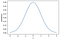Gaussian PDF
• CDF (Cumulative Density Function) calculates the cumulative likelihood for the observation and all prior observations in the sample space. Cumulative density function is a plot that goes from 0 to 1.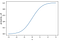Gaussian CDF
• PMF (Probability Mass Function) is a function that gives the probability that a discrete random variable is exactly equal to some value. It differs from a PDF because the latter is associated with continuous random variables and it needs to be integrated over an interval in order to yield a probability. We therefore refer to PMF when touching upon discrete distributions — in this case — Bernoulli, Binomial, and Geometric.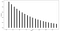Probability Mass Function for Geometric Distribution with p=0.1

There are other probability functions in statistics and a more in-depth explanation can be found here: https://www.itl.nist.gov/div898/handbook/eda/section3/eda362.htm.

# Gaussian Distribution aka Normal Distribution

Among all the distributions we see in practice the Gaussian distribution is the most common. Variables such as SAT scores and heights of female/male adult follow this distribution. The normal distribution always describes a symmetric, unimodal, bell-shaped curve. The distribution depends on two parameters, 𝑢 and 𝜎, therefore we can write it as 𝑁(𝜇,𝜎). When 𝜇=0 and 𝜎=1, then in that case we talk about Standard Normal distribution.

The probability density function of the Normal Distribution is somewhat a complex formula: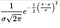Probability Density Function for Normal Distribution

Luckily for us we can refer to it through some tables with values depending on parameters 𝑢 and 𝜎, or using R or Python. Below a Python snippet you can use in order to create a Normal Distribution with 𝑢=0 and 𝜎=1.Gaussian PDFGaussian CDF

How many people have an SAT score below Simone’s score of 1300 if population have μ=1100 and σ = 200?

You can solve this using `scipy.stats` in python:

`from scipy.stats import normp_value = norm.cdf(x=1300, loc=1100, scale=200); p_value# Output -> 0.8413447460685429`

You can also solve the above question in a different manner using the standardised Z-score. How to achieve that? Using the formula to find Z: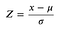Z-score

Z = (x-𝜇)/𝜎 = (1300–1100) / 200 = 1
Now you need to find out the probability distribution associated with Z=1. You can use either some pre-calculated tables or Python (or R). With Python you can use the following snippet:

`from scipy.stats import norm# We append `1-` because we are looking at the right tail.1 - norm.sf(abs(Z_score_you_found))# output = 0.84`

# Student’s t-Distribution

Student distribution is used to estimating the mean of a normally-distributed population in situations where the sample size is small and the population’s standard deviation is unknown. The t-distribution is symmetric and bell-shaped, like the Normal distribution, however it has heavier tails, meaning it is more prone to producing values that fall far from its mean. t-distribution is characterised by the degrees of freedom which equals to 𝜈=𝑛−1 (𝜈 is pronounced as nu).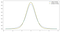t-distribution at different degree of freedom

As you can see from the graph above, the bigger the degree of freedom, the slimmer are the tails. This also means that with 𝜈 increasing the t-distribution approximates more and more to the Normal distribution. More on the subject at the following link: https://mathworld.wolfram.com/Studentst-Distribution.html.

# Geometric Distribution

How many visits should we expect from a user before making a purchase? How long should we expect to flip a coin until it turns up heads? These can be expressed through a Geometric Distribution.

Each trial has two possible outcomes, purchase or not purchase for the first example, and heads or tails in the second. This is represented through a Bernoulli Distribution in statistics. Let’s first see what Bernoulli defines.

If X is a random variable that takes value 1 with probability of success p and 0 with probability 1-p, then X is a Bernoulli random variable with mean and standard deviation as follow: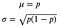Bernoulli-Mean and Standard Deviation

Suppose, on a specific e-commerce page a user make a purchase with probability 10%, based on historical data. Each user can be thought as a trial. The probability of success in this case is p = 0.1, whereas the probability of a failure is denoted as q = 1 — p = 0.9.

If the probability of a success in one trial is p and the probability of a failure is 1 — p, then the probability of finding the first success in the n(th) trial is as follow: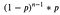Probability in Geometric Distribution

And expected value, variance, and standard deviation are:Mean / Expected Value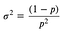Variance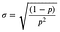Standard Deviation

You may be interested on creating a series of random variates based on the parameters of your distribution. In our previous example, we assumed that the probability of converting for a user was p = 0.1. You can use Python to create those variates:

`from scipy.stats import geomgeom.rvs(0.1, size=10)# Output # array([10,  9,  8,  3,  2,  9,  4, 14, 13,  4])`

If you are interested on plotting the probability mass function (because it is a discrete random variable) for the distribution with parameter p = 0.1, then you can to use the following snippet:

`# 0 to 20 usersx = np.arange(0, 20)# Define the probability for each userpmf = geom.pmf(x, p=0.1)plt.figure(figsize=(15,8))plt.vlines(x ,0, pmf, lw=8)plt.ylabel(‘Probability’)plt.xlabel(‘Users’);`Probability Mass Function for Geometric Distribution with p=0.1

The cumulative density function for the same example above is represented by:

`# 0 to 20 usersx = np.arange(0, 20)# Define the probability for each usercdf = geom.cdf(x, p=0.1)plt.figure(figsize=(15,8))plt.vlines(x, 0, cdf, lw=8)plt.ylabel('Probability')plt.xlabel('Users');`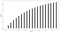Cumulative Density Function for Geometric Distribution with p=0.1

As represented above, the cumulative density function increase step by step. Indeed it is simply the sum of all the previous probability until that point (the sum of each probability of the PMF till that point to be precise). This also means that the cumulative probability of a user making a purchase gets bigger users after users. E.g. if we know that our users make a purchase with p = 0.1, we know that we expect to drive a purchase within around 10 users — on average — if you are far off from it…well, you better have a look at your last release :).

What is the probability that the 5th user landing on the e-commerce page today will make a purchase? Probability of a user converting, based on historical data, p=0.1.

`from scipy.stats import geomgeom.pmf(5, p=0.1)# Output -> 0.06561`

What is the probability that a user will make a purchase within the next 7 trials/users visiting the e-commerce page?

`from scipy.stats import geomgeom.cdf(7, p=0.1)# Output -> 0.5217031`

# Binomial Distribution

The Binomial Distribution is used to describe the number of success in a fixed number of trials. This is different from the geometric distribution, which describes the number of trials we must wait before we observe a success. We need to check that four conditions are respected first:

1. Trials are independent.
2. The number of trials, n, is fixed.
3. Each trial outcome can be classified as a success or failure.
4. The probability of a success, p, is the same for each trial.

Suppose the probability of a singe trial being a success is p. Then the probability of observing exactly k success in n independent trials is given by: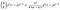Probability in Binomial Distribution

Mean, variance and standard deviation are: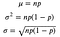Binomial Distribution

With it we can understand what is the probability of having 1, 2, 3 purchases from our users landing in the e-commerce page.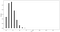Binomial Distribution’s PMF Plot with: p=0.1, n=20

Based on the previous example, regarding the probability of a user purchasing in an e-commerce page.

What is the probability of 2 users making a purchase out of 20 users landing on the page? p=0.1, k= 2, n=20.

You could infer it from the graph above, it is around 25%, but if you want to have a precise value you can calculate it directly with python:

`from scipy.stats import binombinom.pmf(k=2, p=0.1, n=20)# Output -> 0.28518`

What is the probability of hiring 2 persons out of 50 candidates if you know that on average your company hire 1 out of 50 candidates?

`from scipy.stats import binombinom.pmf(k=2, p=0.02, n=50)# Output -> 0.19`

Note: The binomial distribution with probability of success p is nearly normal when the sample size n is sufficiently large that np and n(1-p) are both at least 10. This means we calculate our expected value and standard deviation: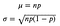Binomial Distribution

And after we find Z, and we can check its probability (as showed at the beginning of the article):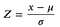Binomial Approximate to Normal under certain conditions

# Poisson Distribution

The Poisson distribution is often useful for estimating the number of events in a large population over a unit of time. In general for applying Poisson the events need to be independent, the average rate (event per time period) is constant, and two events cannot occur at the same time. The average rate is 𝜆 (lambda). Using the rate, we can describe the probability of observing exactly k events in a unit of time.

Following a Poisson distribution are variables like visitors on a website, customer calling an help center, movements in stock price. For example, if you want to know the how many users will land on a page in the next 60 seconds, that can be modelled by a Poisson distribution and the PMF describing it is as follow: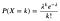Poisson PMF

If you want to know what is the probability of observing 55 users in the next 60 second, when 𝜆 = 45/m (45 users per minute), then:

`poisson.pmf(mu=45, k=55)# Output -> 0.01904389862124531`

If you want to know the probability of observing more than 55 users in the next 60 seconds, when 𝜆 = 45/m (45 users per minute):

`1 - poisson.cdf(mu=45, k=55)# Output -> 0.06255849601658914`

What is the probability of hiring 2 persons out of 60 candidates if you have p=0.02?

`# You could opt to use a Poisson in this way:p = 0.02mu = p * 60  # 1.2poisson.pmf(mu = mu, k = 2)# Output -> 0.22# Or, easier, a Binomialbinom.pmf(k=2, p=0.02, n=60)# Output -> 0.22`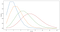Poisson at Various Rates

# Lognormal Distribution

A lognormal distribution is a probability distribution with a normally distributed logarithm. Right skewed distributions with low mean values, large variance, and all positive values often fit this distribution. Example of lognormal distribution in nature are the amount of rainfall, milk production by cows, and for most natural growth processes, where the growth rate is independent of size.

Great resource: https://brilliant.org/wiki/log-normal-distribution/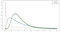Lognormal graph with various parameters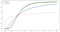Lognormal CDF graph with various parameters

Data Analyst